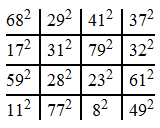# Magic squares of squares

In 1770, Leonhard Euler sent this particular curiosity to Joseph Lagrange. It’s a 4-by-4 magic square, all of whose entries are perfect squares.Martin Gardner offered a prize for finding a 3-by-3 magic square of squares. Lee Sallows found a weaker version where all rows, columns and one of the diagonals have the same sum, but no-one has found either an example or a proof of the non-existence of fully magic squares of squares. If we relax the condition that all entries are distinct, the problem becomes trivial:These trivial examples correspond to solutions of the Diophantine equation$a^2 + b^2 = 2c^2$, which are in turn equivalent to rational points on the circle with radius$\sqrt{2}$. Once you have one solution (such as$a = b = c$), then it is possible to find all of the solutions by considering lines of rational slope passing through this point.

If we allow larger magic squares, there exist magic squares which remain magic when every element is squared. These are known as bimagic squares, the smallest known example having a side length of 8, a sum of 260 and a sum of squares of 11180.

Returning to the original problem of finding a 3-by-3 magic square of squares, it is not too difficult to demonstrate equivalence to the problem of solving a bunch of simultaneous quadratic Diophantine equations. Another problem that can be stated in this form is the difficulty of finding a perfect cuboid: does there exist a cuboid such that every pair of vertices has an integer distance of separation?

This entry was posted in Uncategorized. Bookmark the permalink.

### 0 Responses to Magic squares of squares

1.Dawit says:

I have the proofs for none existence of perfect cuboid and perfect squres of magic squres. But I don’t know what I have to do. If any one can help me in all the activites of posting the proofs.